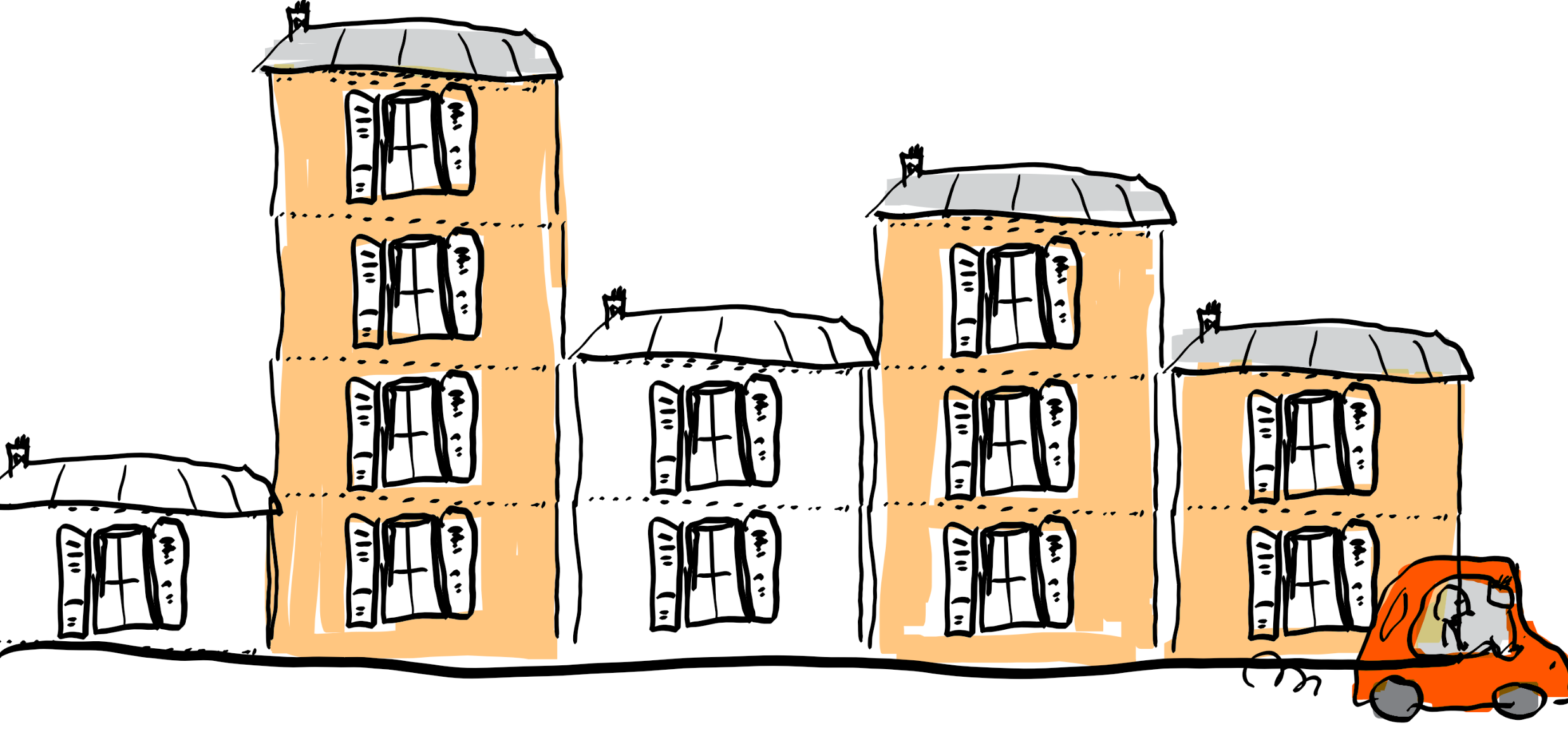Given an array $x$ and an integer $k$, determine for every index $1 \leq j\leq n$ the maximum $x[i]$ among all indices $\max\{1,j-k+1 \} \leq i \leq j$.

# Use a double ended queue

The high level idea is to maintain a window over $x$, ranging from index $j-k+1$ to index $j$. We say that in this range, index $b$ dominates index $a$ if $a < b$ and $x[a] \leq x[b]$.

For an intuitive explanation, imagine that on a coastline, there are $n$ building standing in a row. Each building has the same width, which we call a block. Building $i$ (numbered from left to right) consists of $x[i]$ stories. You drive a car on the coast line left to right and look in your rear mirror. Due to the fog, you can’t see buildings that are more than $k$ blocks far. In addition, any building in some block $b$ will hide all smaller or equal height buildings in blocks $a$ to the left of $b$. This is meant by index $b$ dominating index $a$.Instead of maintaining the actual window, we maintain in a queue $S$ all non-dominated indices in the window. The queue contains indices in increasing order, and their corresponding $x$-values decrease in this order. Hence $S$ is the index of the largest value in the window, and $S[-1]$ is the index of the last value which entered the window.

When sliding the window $[i,j]$, $x[j]$ enters the window, while $x[i]$ leaves the windows. If $x[i]$ was the maximum in the window, i.e. $S=i$, then $i$ has to be removed from $S$. And before $j$ enters $S$, we need to remove all indices dominated by $j$.

We use a double ended queue, because we need to remove dominated elements from the right, insert new elements to the right, and remove the maximum element from the left.

Note that when $j$ ranges over the first $k$ indices, no element leaves $S$ yet, since the window does not have size $k$ yet.

For the time complexity, note that every element might enter and leave $S$ at most once, hence the work done on every index is constant in amortized time, and the overall complexity is $O(n)$, where $n$ is the size of $x$.